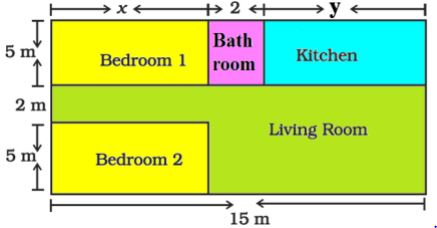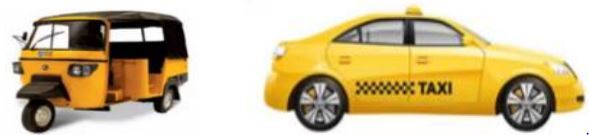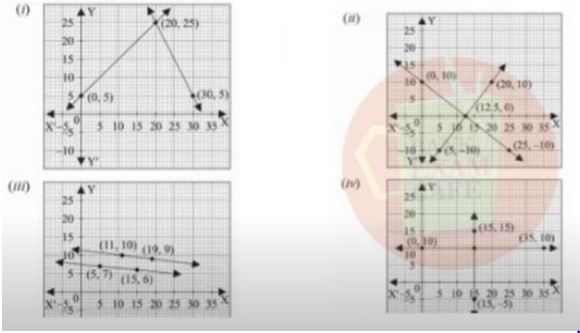# CBSE Board Exam 2021: Check Case Study Based Question Bank for Class 10 Maths Chapter 3 (Released By CBSE)

CBSE's question bank on case study for Class 10 Maths Chapter 3 is available here. These questions will be very helpful to prepare for the CBSE Class 10 Maths Exam 2021.

Created On: Apr 7, 2021 19:14 ISTCBSE Class 10 Maths Case Study Based Questions for Chapter 3

CBSE Board Exam 2021: Case study based questions in Maths are new for class 10 students as the board has introduced these questions for the first time in the Maths sample paper. CBSE Class 10 Maths Question Paper 2021 will include four questions on case study in Part A (containing objective type questions). To help students understand the format of the questions and they can be answered, CBSE has released a question bank on case study for class 10 Maths. Students must practice with these questions to get familiarised with the concepts and logic used in the case study and understand how to answers them correctly. You may check below the case study questions for CBSE Class 10 Maths Chapter 3 - Pair of Linear Equations in Two Variables. You can also check the right answer at the end of each question.

Check Case Study Questions for Class 10 Maths Chapter 3 - Pair of Linear Equations in Two Variables

CASE STUDY-1:

A test consists of ‘True’ or ‘False’ questions. One mark is awarded for every correct answer while ¼ mark is deducted for every wrong answer. A student knew answers to some of the questions. Rest of the questions he attempted by guessing. He answered 120 questions and got 90 marks.

 Type of Question Marks given for correct answer Marks deducted for wrong answer True/False 1 0.25

1. If answer to all questions he attempted by guessing were wrong, then how many questions did he answer correctly?

2. How many questions did he guess?

3. If answer to all questions he attempted by guessing were wrong and answered 80 correctly, then how many marks he got?

4. If answer to all questions he attempted by guessing were wrong, then how many questions answered correctly to score 95 marks?

Answers:

Let the no of questions whose answer is known to the student x and questions attempted by cheating be y

x + y =120

x – 1/4y =90

solving these two

x = 96 and y = 24

1. He answered 96 questions correctly.

2. He attempted 24 questions by guessing.

3. Marks = 80- ¼ 0f 40 =70

4. x – 1/4 of (120 – x) = 95

5x = 500, x = 100

CASE STUDY-2:

Amit is planning to buy a house and the layout is given below. The design and the measurement has been made such that areas of two bedrooms and kitchen together is 95 sq.m.Based on the above information, answer the following questions:

1. Form the pair of linear equations in two variables from this situation.

2. Find the length of the outer boundary of the layout.

3. Find the area of each bedroom and kitchen in the layout.

4. Find the area of living room in the layout.

5. Find the cost of laying tiles in kitchen at the rate of Rs. 50 per sq.m.

ANSWER:

1. Area of two bedrooms= 10x sq.m

Area of kitchen = 5y sq.m

10x + 5y = 95

2x + y =19

Also, x + 2+ y = 15

x + y = 13

2. Length of outer boundary = 12 + 15 + 12 + 15 = 54m

3. On solving two equation part(i)

x = 6m and y = 7m

area of bedroom = 5 x 6 = 30m

area of kitchen = 5 x 7 = 35m

4. Area of living room = (15 x 7) – 30 = 105 – 30 = 75 sq.m

5. Total cost of laying tiles in the kitchen = Rs50 x 35 = Rs1750

Case study-3:

It is common that Governments revise travel fares from time to time based on various factors such as inflation ( a general increase in prices and fall in the purchasing value of money) on different types of vehicles like auto, Rickshaws, taxis, Radio cab etc. The auto charges in a city comprise of a fixed charge together with the charge for the distance covered. Study the following situations:Name of the city Distance travelled (Km) Amount paid (Rs.) City A 10 75 15 110 City B 8 91 14 145

Situation 1: In city A, for a journey of 10 km, the charge paid is Rs 75 and for a journey of 15 km, the charge paid is Rs 110.

Situation 2: In a city B, for a journey of 8km, the charge paid is Rs91 and for a journey of 14km, the charge paid is Rs 145.

Refer situation 1

1. If the fixed charges of auto rickshaw be Rs x and the running charges be Rs y km/hr, the pair of linear equations representing the situation is

a) x + 10y =110, x + 15y = 75

b) x + 10y = 75, x + 15y = 110

c) 10x + y = 110, 15x + y = 75

d) 10x + y = 75, 15x + y = 110

Answer: b) x + 10y = 75, x + 15y = 110

2. A person travels a distance of 50km. The amount he has to pay is

a) Rs.155

b) Rs.255

c) Rs.355

d) Rs.455

Answer: c) Rs.355

Refer situation 2

3. What will a person have to pay for travelling a distance of 30km?

a) Rs.185

b) Rs.289

c) Rs.275

d) Rs.305

Answer: b) Rs.289

4. The graph of lines representing the conditions are: (situation 2)Answer: (iii)

Comment ()

8 + 1 =
Post